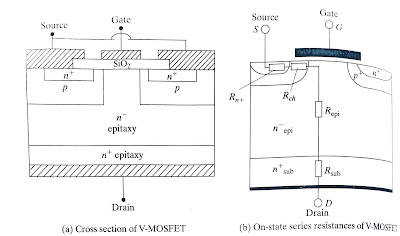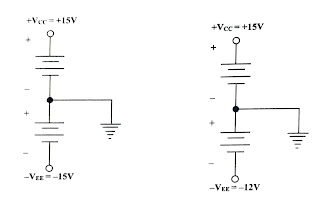Ideal op-amp. It has two input signals V₁ and V2. applied to non-inverting and inverting terminals respectively. The following things can be observed for the ideal op-amp. An ideal op-amp draws no current at both the input terminals i.e. I₁ = I=0 thus the input impedance is infinite.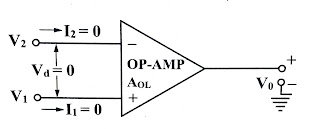The gain of the ideal op-amp is infinite hence the differential input V-V₁-V₂ is essentially the zero for the finite output voltage V₁.

The output voltage V0, is independent of the current drawn from the output terminals. Thus the output impedance is zero and hence can drive an infinite number of other circuits.

The various characteristics of ideal op-amp are

Infinite voltage gain: it is denoted as AoL. It is the differential open loop gain and is infinite for an ideal op-amp.

Infinite input impedance: the input impedance is denoted as Rin, and is infinite for an ideal op-amp. This ensures that no current can flow into as ideal op-amp.

Zero output impedance: The output impedance is denoted as R0. and is zero for an ideal op-amp. This ensures that the output voltage of the op-amp remains same, irrespective of the value of the load resistance connected.

Zero offset voltage: The presence of the small output voltage through V₁=V₂=0 is called an offset voltage. It is zero for an ideal op-amp. This ensures zero output for zero input signal voltage.

Infinite Band width: The range of frequency over which the amplifier performance is satisfactory is called its bandwidth. The band width of an ideal op-amp is infinite. This means that operating frequency range is 0 to .

Infinite CMRR: The ratio of differential gain and common mode gain is defined as CMRR. Thus infinite CMRR of an ideal op-amp ensures zero common mode gain. Due to this common mode noise output voltage is zero for an ideal op-amp.

Infinite slew rate: This ensures that the changes in the output voltage occur simultaneously with the change in the input voltage.

Infinite slew rate indicates that output changes simultaneously with the time in the input voltage.

The parameter slew rate is actually defined as the maximum rate of change of output voltage with time and expressed in V/μs.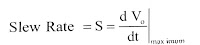No-effect of temperature: The characteristics of op-amp do not change with temperature.

Power Supply Rejection Ratio: The power supply rejection ratio is defined as the ratio of the change in input offset voltage due to the change in supply voltage producing it, keeping the other power supply voltage constant. It is also called as power supply sensitivity.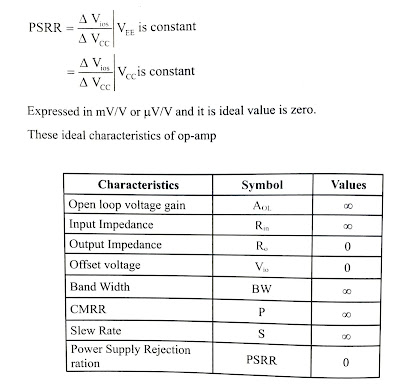See More: